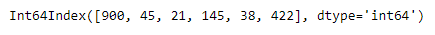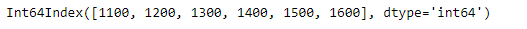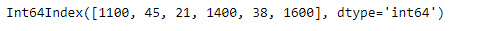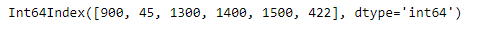Skip to content
Related Articles
Python | Pandas Index.where
• Last Updated : 20 Feb, 2019

Pandas Index is an immutable ndarray implementing an ordered, sliceable set. It is the basic object which stores the axis labels for all pandas objects.

Pandas` Index.where` function return an Index of same shape as self and whose corresponding entries are from self where cond is True and otherwise are from other.

Syntax: Index.where(cond, other=None)

Parameter :
cond : boolean array-like with the same length as self
other : scalar, or array-like

Returns : Index

Example #1: Use `Index.where` function to return an Index, in which we select the value from the other Index if value of this Index is not smaller than 100.

 `# importing pandas as pd``import` `pandas as pd`` ` `# Creating the first index``idx1 ``=` `pd.Index([``900``, ``45``, ``21``, ``145``, ``38``, ``422``])`` ` `# Creating the second index``idx2 ``=` `pd.Index([``1100``, ``1200``, ``1300``, ``1400``, ``1500``, ``1600``])`` ` `# Print the first index``print``(idx1)`` ` `# Print the second index``print``(idx2)`

Output :Now we will use `Index.where` function to return an Index, in which we select the value from the other Index if value of this Index is not smaller than 100.

 `# return the new index based on the condition``result ``=` `idx1.where(idx1 < ``100``, idx2)`` ` `# Print the result``print``(result)`

Output :As we can see in the output, the `Index.where` function has successfully returned an Index object satisfying the passed condition.

Example #2 : Use `Index.where` function to return an Index, which satisfy the passed condition.

 `# importing pandas as pd``import` `pandas as pd`` ` `# Creating the first index``idx1 ``=` `pd.Index([``900``, ``45``, ``21``, ``145``, ``38``, ``422``])`` ` `# Creating the second index``idx2 ``=` `pd.Index([``1100``, ``1200``, ``1300``, ``1400``, ``1500``, ``1600``])`` ` `# Print the first index``print``(idx1)`` ` `# Print the second index``print``(idx2)`

Output :Now we will use `Index.where` function to return an Index, in which we select the value from the other Index if value of other Index minus 1200 is not smaller than idx1.

 `# return the new index based on the condition``result ``=` `idx1.where((idx2 ``-` `1200``) < idx1, idx2)`` ` `# Print the result``print``(result)`

Output :As we can see in the output, the `Index.where` function has successfully returned an Index object satisfying the passed condition.

Attention geek! Strengthen your foundations with the Python Programming Foundation Course and learn the basics.

To begin with, your interview preparations Enhance your Data Structures concepts with the Python DS Course. And to begin with your Machine Learning Journey, join the Machine Learning – Basic Level Course

My Personal Notes arrow_drop_up